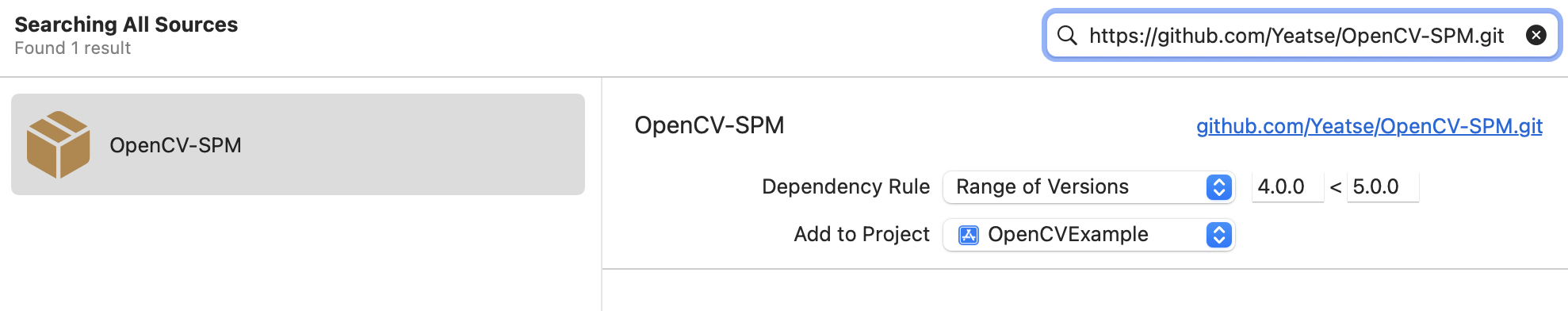# Swiftpack.co - Yeatse/OpenCV-SPM as Swift Package

Swiftpack.co is a collection of thousands of indexed Swift packages. Search packages.
Yeatse/OpenCV-SPM 4.6.0
A swift package wrapping around OpenCV
⭐️ 1
🕓 1 week ago
iOS macOS macCatalyst
.package(url: "https://github.com/Yeatse/OpenCV-SPM.git", from: "4.6.0")

# OpenCV-SPM

Use OpenCV in your Swift project in a more elegant way.

This swift package helps you easily import the prebuilt `opencv2.xcframework` into your project, so you no longer need to build it by yourself. It watches the release events in OpenCV Github Project and automatically creates new releases, using the powerful Github Actions.

## Installation

1. Add `https://github.com/Yeatse/OpenCV-SPM.git` to your package dependencies.2. Add `libc++` to your linked libraries, otherwise building will fail.3. Add `-all_load` to `Other Linker Flags`, otherwise some methods cannot be used. opencv/opencv#17532## Usage

Import `opencv2` and use it as documented in opencv.org. For example, a swift version of Extract horizontal and vertical lines by using morphological operations:

``````import opencv2

// Show source image
let src = Mat(uiImage: image)

// Transform source image to gray if it is not already
let gray: Mat
if (src.channels() == 3) {
gray = Mat()
Imgproc.cvtColor(src: src, dst: gray, code: .COLOR_BGR2GRAY)
} else {
gray = src
}

// Apply adaptiveThreshold at the bitwise_not of gray, notice the ~ symbol
let notGray = Mat()
Core.bitwise_not(src: gray, dst: notGray)

let bw = Mat()
Imgproc.adaptiveThreshold(src: notGray, dst: bw, maxValue: 255, adaptiveMethod: .ADAPTIVE_THRESH_MEAN_C, thresholdType: .THRESH_BINARY, blockSize: 15, C: -2)

// Create the images that will use to extract the horizontal lines
let horizontal = bw.clone()
let vertical = bw.clone()

// Specify size on horizontal axis
let horizontalSize = horizontal.cols() / 30
// Create structure element for extracting horizontal lines through morphology operations
let horizontalStructure = Imgproc.getStructuringElement(shape: .MORPH_RECT, ksize: .init(width: horizontalSize, height: 1))
// Apply morphology operations
Imgproc.erode(src: horizontal, dst: horizontal, kernel: horizontalStructure, anchor: .init(x: -1, y: -1))
Imgproc.dilate(src: horizontal, dst: horizontal, kernel: horizontalStructure, anchor: .init(x: -1, y: -1))

// Specify size on vertical axis
let verticalSize = vertical.rows() / 30

// Create structure element for extracting vertical lines through morphology operations
let verticalStructure = Imgproc.getStructuringElement(shape: .MORPH_RECT, ksize: .init(width: 1, height: verticalSize))

// Apply morphology operations
Imgproc.erode(src: vertical, dst: vertical, kernel: verticalStructure, anchor: .init(x: -1, y: -1))
Imgproc.dilate(src: vertical, dst: vertical, kernel: verticalStructure, anchor: .init(x: -1, y: -1))

// Inverse vertical image
Core.bitwise_not(src: vertical, dst: vertical)

// Extract edges and smooth image according to the logic
// 1. extract edges
// 2. dilate(edges)
// 3. src.copyTo(smooth)
// 4. blur smooth img
// 5. smooth.copyTo(src, edges)
// Step 1
let edges = Mat();
Imgproc.adaptiveThreshold(src: vertical, dst: edges, maxValue: 255, adaptiveMethod: .ADAPTIVE_THRESH_MEAN_C, thresholdType: .THRESH_BINARY, blockSize: 3, C: -2)

// Step 2
let kernel = Mat.ones(rows: 2, cols: 2, type: CvType.CV_8UC1)
Imgproc.dilate(src: edges, dst: edges, kernel: kernel)

// Step 3
let smooth = Mat();
vertical.copy(to: smooth)

// Step 4
Imgproc.blur(src: smooth, dst: smooth, ksize: .init(width: 2, height: 2))

// Step 5
smooth.copy(to: vertical, mask: edges)

// Show final result
let result = vertical.toUIImage()
``````

### GitHub

 link Stars: 1 Last commit: 1 week agoSomething's broken? Yell at me @ptrpavlik. Praise and feedback (and money) is also welcome.

### Release Notes

4.6.0
1 week ago

Swiftpack is being maintained by Petr Pavlik | @ptrpavlik | @swiftpackco | API | Analytics